﻿ New Squaring and Square-rooting Circuits Using Cdba

### New Squaring and Square-rooting Circuits Using Cdba

J. K. Pathak, A. K. Singh, Raj SenaniOPEN ACCESSPEER-REVIEWED

## New Squaring and Square-rooting Circuits Using Cdba

J. K. Pathak1, A. K. Singh2, Raj Senani3,1Department of Electronics and Communication Engineering, Echelon Institute of Technology, Faridabad, India

2Department of Electronics and Communication Engineering, Faculty of Engineering and Technology, Sharda University, Ghaziabad, India

3Division of Electronics and Communication Engineering, Netaji Subhas Institute of Technology, Sector 3, Dwarka, New Delhi, India

### Abstract

One new squaring and two new square-rooting circuits based on CDBAs have been introduced. The new squarer circuit consists of a CDBA, two NMOS transistors and one resistor. On the other hand, the first square-rooting circuit consists of two CDBAs, two NMOS transistors while the second square- rooting circuit consists of only a single CDBA, two NMOS transistors and two PMOS transistors. The proposed circuits exhibit wide input voltage range, very small error and offer low output impedance to facilitate easy cascading without requiring additional buffers. PSPICE simulation results are included which confirm the practical workability of the new circuits.

### At a glance: Figures

1234
Prev Next

• Pathak, J. K., A. K. Singh, and Raj Senani. "New Squaring and Square-rooting Circuits Using Cdba." American Journal of Electrical and Electronic Engineering 2.6 (2014): 175-179.
• Pathak, J. K. , Singh, A. K. , & Senani, R. (2014). New Squaring and Square-rooting Circuits Using Cdba. American Journal of Electrical and Electronic Engineering, 2(6), 175-179.
• Pathak, J. K., A. K. Singh, and Raj Senani. "New Squaring and Square-rooting Circuits Using Cdba." American Journal of Electrical and Electronic Engineering 2, no. 6 (2014): 175-179.

 Import into BibTeX Import into EndNote Import into RefMan Import into RefWorks

### 1. Introduction

Squaring and square-rooting circuits are widely used for continuous-time signal processing in measurement and instrumentation systems . In order to maintain compatibility with existing voltage processing circuits as well as taking advantages  of the current-mode circuits, the new active element called Current differencing buffered amplifier (CDBA)  has received significant attention in literature since it can operate in both current-mode as well as voltage- mode. In earlier literature, the CDBA has been shown to offer a lot of flexibility in linear analog circuit design [3, 4, 5, 6]. However, the use of CDBA in realizing non-linear functions has rather been limited [7, 8, 9].

The purpose of this paper is to present new squaring and square-rooting circuits based on CDBAs. The proportionality constant of the new circuits can be controlled by the value of an external resistor. The effectiveness of the proposed circuits is verified through SPICE simulations.

### 2. The Current-differencing Buffered Amplifier (CDBA)

A practical CDBA can be described by the following relationships that take into account the various non-idealities: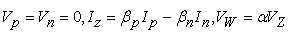(1)

where βp=1-εp and εp( lεpl<<1) is the current tracking error from p-terminal to z-terminal, βn=1-εn and εn( lεnl<<1) is the current tracking error from n-terminal to z-terminal, and α = 1- εv and εv( lεvl<<1) is the voltage-tracking error from z-terminal to w-terminal of the CDBA. The circuit symbol of the CDBA is shown in Figure 1.

### 3. Squarer Circuit

Figure 2 shows the proposed squarer circuit. Assuming matched MOS transistors operating in triode region, a straight forward analysis of the circuit gives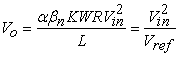(2)

where Vref is the proportionality constant, (W/L) is the aspect ratio of MOS transistors, K=µnCox is the process trans-conductance parameter of the MOS transistor. The proportionality constant Vref can be controlled by resistor R.

The output current Io is given by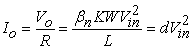(3)

where d is the proportionality constant. Thus, the circuit can work in trans-admittance mode also.

### 4. Square-rooting Circuits

Figure 3 shows the first proposed square-rooting circuit. Assuming MOS transistors M1 and M2 to be matched and operating in triode region, the output voltage is found to be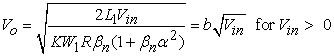(4)

where b is the proportionality constant, (W/L) is the aspect ratio of MOS transistors, K=µnCox is the process trans-conductance of the MOS transistor and the proportionality constant b can be controlled by the resistor R. It is interesting to point out that the same circuit can work for the negative value of Vin if we connect R to p-terminal instead of n-terminal of the CDBA.

In the derivation of equation (4), to enable the cancellation of the non-linear terms, L1=L2 and W22βn2W1 have been taken where (W1/L1) and (W2/L2) are the aspect ratios of the MOS transistors M1 and M2 respectively.

Figure 4 shows the second proposed square-rooting in which the MOS transistors M1 and M2 are operating in triode region and are used to obtain the basic square-rooting function. The MOS transistors M3 and M4 are also operating in the triode region and are used to realize floating voltage controlled resistor . After taking the model parameters of the CMOS process from , Vg=2.63V, L1=L2, W1=0.2455W2, a routine analysis of the proposed circuit then gives the following expression for its output voltage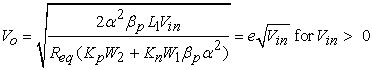(5)

where e is the proportionality constant, (W1/L1) and (W2/L2) are the aspect ratios of MOS transistors M1 and M2 respectively, KnnCox and KppCox being the process transconductances of the NMOS and PMOS transistor respectively.

Here, the proportionality constant e can be controlled by the resistor Req which is given by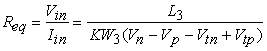(6)

where (W3/L3) is the aspect ratio of the MOS transistor M3, Vn and Vp are the gate voltages of M3 and M4 respectively, Vtn and Vtp are the threshold voltage of M3 and M4 respectively. To cancel non-linear terms, W4 is taken equal to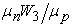.

The same circuit can work for the negative value of Vin if we connect the CMOS transistors constituting Req to the n-terminal of the CDBA instead of the p-terminal.

### 5. SPICE Simulation Results

To verify the theoretical results, the squarer circuit has been simulated in SPICE using the CDBA from  operating at ± 5V DC supply with 0.35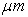CMOS process parameters. The value of R is made equivalent to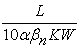so that proportionality constant becomes equal to 10.

Using SPICE, the values of α, βp and βn of the CDBA were found to be 1.0000998, 1.002501 and 1.002501 respectively. With L1=L2=28, W1=W2=35, the value of R, to make proportionality constant equal to 10, has been adjusted to 990Ω. Figure 5 shows the DC transfer characteristics of the proposed squarer circuit. Figure 6 shows the transient response of the circuit to a sinusoidal input of 4Vpp, having zero average value and frequency of 1 kHz.

The square rooting circuit 1 of Figure 3 has been simulated in SPICE using the CDBA  operating at +5 Volts DC supply with 0.35CMOS process parameters. In the simulations the value of R is made equivalent to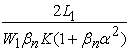so that the proportionality constant b becomes equal to 1. To realize unity gain inverting amplifier, a CDBA-based inverter has been used. By selecting L1=L2=21, W1=21then W22βn2W1 comes out to be approximately equal to 21. To get unity proportionality constant b, the value of R was adjusted to 12 kΩ. Figure 7 shows the DC transfer characteristics of the proposed square-rooting circuit. Figure 8 shows the transient response of the circuit to a sinusoidal input of 1Vpp, average of 0 Volts and frequency of 1 kHz.

In the simulations of the square rooting circuit 2 of Figure 4, CDBA from  was used operating at ± 12V DC supply with the value of Req made equivalent to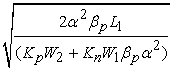so that proportionality constant e becomes equal to 1. By selecting L1=L2=24, W2=40then W1=0.2455W2 comes out to be equal to 81.45. To obtain unity proportionality constant, the value of Req was adjusted to 8722Ω by selecting L3=L4=67, W3=15, W4=57.11, Vp = 4V and Vn = -4V.The circuit of Figure 4 was also simulated using the same aspect ratios of MOS transistors and adjusted value of Req. Figure 9 shows the DC transfer characteristics of the square rooting circuit. Figure 10 shows the transient response of the circuit to a sinusoidal input of 1Vpp, average of 0.5V and frequency of 1 kHz. Apart from the DC transfer characteristics and transient response of the proposed circuits, Table 1 shows the input voltage range, % error for this range and the band width of the proposed circuits.

Figure 10. Output voltage waveform obtained from the square rooting circuit 2 for 1 KHz sinusoidal input

#### Table 1. Input voltage range, % error and band width of the proposed circuitsDownload asPowerPoint Slide

Veiw figureView current table in a new window

### 6. Comparison with the Existing CDBA-Based Squarer and Square Rooting Circuits

Squarer circuit can also be realized from the four quadrant multiplier proposed by Keskin in  by taking two input signals to be same but such a realization will need an additional summer and difference circuits also. By contrast, in the proposed squarer circuit, only an additional inversion circuit is required. On the other hand, the current-mode squarer proposed by Lawanwisut and Siripruchyanun  needs four current-controlled CDBAs against one as required by the proposed squarer circuit. Furthermore, no square-rooting circuit has been reported earlier using CDBAs.

As compared to the squarer circuit based on Current Conveyors (CC) , the proposed squarer circuit has low output impedance to facilitate easy cascading without the need of additional buffers. Also, the band width of the proposed square-rooting circuits is 3.46 MHz as compared to 400 kHz for the CC-based square-rooting circuit of . Furthermore, the proposed square-rooting circuit 1 employs only two active elements and the square-rooting circuit 2 employs only one active element as compared to three active elements (one CCII+ and two op-amps) used in the CC-based circuits of .

### 7. Concluding Remarks

New squarer and square-rooting circuits based on the CDBA have been proposed. These circuits exhibit wide input voltage range, smaller errors and have a low output impedance to facilitate easy cascading without requiring additional buffers. The proportionality constant of these circuits can be controlled by the value of an external resistor. Squarer circuit also works in trans-admittance mode. SPICE simulations have verified the workability of the proposed circuits.

### Acknowledgement

This work was performed at the Analog Signal Processing Research Lab of NSIT, New Delhi.

### References

  Ismail, M. “Analog VLSI: Signal and Information Processing (International Edition),” McGraw-Hill Inc. 1994.In article  Roberts, G. W. and Sedra. A. S., “All current-mode frequency selective circuits,” Electronics Letters, 2. 759-761. 1989.In article CrossRef  Acar, C. and Ozoguz, S., “A new versatile building block: current differencing buffered amplifier suitable for analog signal processing filters,” Microelectronics Journal, 30. 157-160. 1999.In article CrossRef  Acar, C. and Sedef, H., “Realization of nth-order current transfer function using current-differencing buffered amplifiers,” International Journal of Electronics, 90. 277-283. 2003In article CrossRef  Maheshwari, S. and Khan, I. A., “Current controlled current differencing buffered amplifier: Implementations and Applications,” Active and Passive Electronic Components, 4. 219-227. 2004.In article CrossRef  Pathak, J. K., Singh, A. K. and Senani, R., “Systematic realization of quadrature oscillators using current differencing buffered amplifiers,” IET Circuits, Devices and Systems, 5. 203-211. 2011.In article CrossRef  Keskin, A.U., “A Four quadrant analog multiplier employing single CDBA,” Analog Integrated Circuits and Signal Processing, 40. 99-101. 2004.In article CrossRef  Siripruchyanun, M., “A Design of Analog Multiplier and Divider Using Current Controlled Current Differencing Buffered Amplifiers,” International Symposium on Integrated Circuits, 568-571. 2007.In article  Lawanwisut, S. and Siripruchyanun, M., “Temperature Insensitive Electronically Controllable Current-mode Squarer Based on CC-CDBAs,” Proceedings of the 1st International Conference on Technical Education (ICTE2009), 225-228. 2009.In article  Al-Shahrani, S.M., “CMOS wideband auto-tuning phase shifter circuit,” IET, Electronics Letters, 43. 804-806. 2007.In article CrossRef  Senani, R. and Singh, A. K., “A new Universal Current mode biquad filter”, Frequenz, 56. 55-59. 2002.In article CrossRef  Liu, S.I., “Square-rooting and vector summation circuits using current conveyors,” IEE Proc. Circuits, Devices and Systems. 142. 223-226. 1995.In article